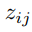# [Paper review] Learning Deep Disentangled Embeddings With the F-Statistic Loss (NeurIPS 2018)…

Source: Deep Learning on Medium

This post is a paper review for educational purpose.

In part 1, we looked at existing metric losses. This time, let’s look at the new metric loss released at NeurIPS 2018. (metric loss refers to the objective loss used in metric learning.)

To put it easily, F-statistic loss is a metric loss that induces a difference in class distribution based on the Fisher–Snedecor (or F) distribution. Let’s take a look at what F-distribution loss is.

In general, the purpose of metric learning is to keep features of other classes away from each other, while keeping features of the same class close to each other. For example, when we want to separate two classes, the distribution is as follows.

Our goal here is to learn to keep the mean values ​​of the two distributions with n_1 and n_2 instances away from each other. Therefore, lowering the probability value below is our main objective. Because the two distributions have the same mean, it is assumed that the distribution of the two classes is difficult to distinguish.

Here, z can be regarded as a coordinate of instance lying on the embedding space, and the definition of z is as follows.Figure 2. Definition of embedding coordinate of instance j of class i.

For example, the second instance of the first class represents z_12.

Since this posterior distribution is difficult to calculate directly (intractable), we can calculate it by converting it to likelihood. (Bayes’ rule is used and the denominator value is omitted because it is a normalize constant.)

Also, s(z) is as follows.

s is simply a ratio of between-class variability to within-class variability. In other words, it is a form of function that fits the objective function we want to learn. Therefore, learning to grow the value of s(z) will also be our primary goal. The average value of the instance is hat z, and the number of instances is tilde n.

To calculate the value of s(z), we can measure the separation of different classes based on the CDF of the F distribution as follows. Please refer to the following link for a detailed description of F distribution. (https://en.wikipedia.org/wiki/F-distribution)

The values ​​of the second and third terms of the I function are in the range of degrees of freedom and can be considered from 1 to the sum of instances of two classes. Since the CDF of the F distribution is a regularized beta function, I can be modeled as a beta function. It is also possible to integrate with neural networks that learn based on gradient optimization because this value is differentiable.

The larger the s value, the larger the degree of class separation. So we need to design the probability of Figure 4 larger.

Finally, when we expand probability of Figure 4 to multi-class, equation is as follows.

Alpha and beta can be regarded as a set of class C elements. Also k represents the dimension of embedding instance.

Therefore, final loss equation of F-statistic loss is as follows.

The reason why the final loss is negative is that it must learn to increase the probability value modeled by the beta function as mentioned above.

Based on the picture below, we can understand the concept of the proposed method more easily. We get z through the neural network based on the data x. In this case, when k = 2, the degree of separation has the maximum value, and in the remaining k except for 2, the loss is learned to be zero.

There are 4 characteristics of F-statistic loss as defined.

1. Even if at least some class separation values ​​are learned among the embedding instances, the gradient decreases very rapidly. (i.e., accurate and fast learning is possible during the learning process.)

2. Unlike other metric losses, it is invariant to rotations.

3. It is easy to optimize with only a few hyper-parameters.

4. Because loss is defined based on probability value, it is easy to integrate with other probabilistic loss such as Kullback–Leibler divergence(KLD) loss of VAE.

Below table represents the results of retrieval task performed in the paper suggesting metric losses. If you want to know more information of measurement, then you can also refer follow link. (https://medium.com/@m_n_malaeb/recall-and-precision-at-k-for-recommender-systems-618483226c54)